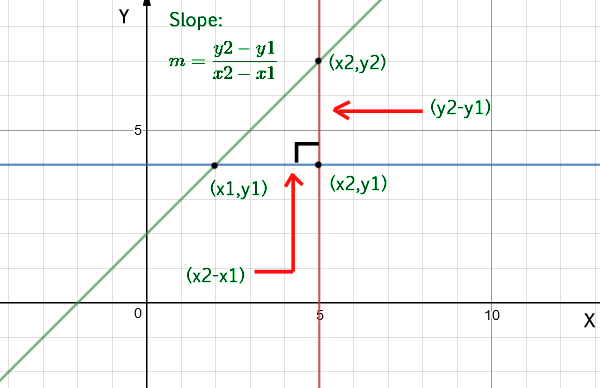# Program to find slope of a line

• Difficulty Level : Easy
• Last Updated : 04 Sep, 2021

Given two coordinates, find the slope of a straight line.

Examples:

```Input  : x1 = 4, y1 = 2,
x2 = 2, y2 = 5
Output : Slope is -1.5```

Approach: To calculate the slope of a line you need only two points from that line, (x1, y1) and (x2, y2). The equation used to calculate the slope from two points is:Below is the implementation of the above approach:

## C++

 `// C++ program for slope of line` `#include ` `using` `namespace` `std;`   `// function to find the slope of a straight line` `float` `slope(``float` `x1, ``float` `y1, ``float` `x2, ``float` `y2)` `{` `    ``return` `(y2 - y1) / (x2 - x1);` `}`   `// driver code to check the above function` `int` `main()` `{` `    ``float` `x1 = 4, y1 = 2;` `    ``float` `x2 = 2, y2 = 5;` `    ``cout << ``"Slope is: "` `         ``<< slope(x1, y1, x2, y2);` `    ``return` `0;` `}`

## Java

 `// Java program for slope of line` `import` `java.io.*;`   `class` `GFG {` `    ``static` `float` `slope(``float` `x1, ``float` `y1,` `                       ``float` `x2, ``float` `y2)` `    ``{` `        ``return` `(y2 - y1) / (x2 - x1);` `    ``}` `    ``public` `static` `void` `main(String[] args)` `    ``{` `        ``float` `x1 = ``4``, y1 = ``2``;` `        ``float` `x2 = ``2``, y2 = ``5``;` `        ``System.out.println(``"Slope is "` `+ ` `                    ``slope(x1, y1, x2, y2));` `    ``}` `}`

## Python

 `# Python program for slope of line` `def` `slope(x1, y1, x2, y2):` `    ``return` `(``float``)(y2``-``y1)``/``(x2``-``x1) `   `# driver code    ` `x1 ``=` `4` `y1 ``=` `2` `x2 ``=` `2` `y2 ``=` `5` `print` `"Slope is :"``, slope(x1, y1, x2, y2)`

## C#

 `// C# program for slope of line` `using` `System;`   `class` `GFG ` `{` `    ``static` `float` `slope(``float` `x1, ``float` `y1,` `                       ``float` `x2, ``float` `y2)` `    ``{` `        ``return` `(y2 - y1) / (x2 - x1);` `    ``}` `    `  `    ``// Driver code` `    ``public` `static` `void` `Main()` `    ``{` `        ``float` `x1 = 4, y1 = 2;` `        ``float` `x2 = 2, y2 = 5;` `        ``Console.WriteLine(``"Slope is "` `+ ` `                    ``slope(x1, y1, x2, y2));` `    ``}` `}`   `// This code is contributed by vt_m.`

## PHP

 ``

## Javascript

 ``

Output

`Slope is: -1.5`

Time Complexity: O(1)

Auxiliary Space: O(1)

Special Case: The above code will throw a runtime error when int the function x1 is equal to x2 (x1==x2). In that case, our denominator will become zero(0). To avoid that condition, we will add a condition in the slope function.

## C++

 `// C++ program for slope of line` `#include ` `using` `namespace` `std;`   `// function to find the slope of a straight line` `float` `slope(``float` `x1, ``float` `y1, ``float` `x2, ``float` `y2)` `{` `    ``if``(x1 == x2)` `        ``return` `INT_MAX;` `    ``return` `(y2 - y1) / (x2 - x1);` `}`   `// driver code to check the above function` `int` `main()` `{` `    ``float` `x1 = 4, y1 = 2;` `    ``float` `x2 = 2, y2 = 5;` `    ``cout << ``"Slope is: "` `         ``<< slope(x1, y1, x2, y2);` `    ``return` `0;` `}`

## Java

 `// Java program for slope of line`   `import` `java.util.*;`   `class` `GFG {`   `    ``// function to find the slope of a straight line` `    ``static` `float` `slope(``float` `x1, ``float` `y1, ``float` `x2, ``float` `y2) {` `        ``if` `(x1 == x2)` `            ``return` `Integer.MAX_VALUE;` `        ``return` `(y2 - y1) / (x2 - x1);` `    ``}`   `    ``// driver code to check the above function` `    ``public` `static` `void` `main(String[] args) {` `        ``float` `x1 = ``4``, y1 = ``2``;` `        ``float` `x2 = ``2``, y2 = ``5``;` `        ``System.out.print(``"Slope is: "` `+ slope(x1, y1, x2, y2));` `    ``}` `}`   `// This code contributed by Rajput-Ji `

## Python3

 `# Python3 program to find slope` `import` `sys`   `# Function to find the slope of a straight line` `def` `slope(x1, y1, x2, y2):` `    `  `    ``if` `x1 ``=``=` `x2:` `        ``return` `(sys.maxsize) ` `        `  `    ``return` `((y2 ``-` `y1) ``/` `(x2 ``-` `x1)) `   `# Driver code` `x1 ``=` `4` `y1 ``=` `2` `x2 ``=` `2` `y2 ``=` `5`   `print``(``"Slope is :"``, slope(``4``, ``2``, ``2``, ``5``))`   `# This code is contributed by vaishaligoyal878`

## C#

 `// C# program for slope of line` `using` `System;` `class` `GFG {`   `    ``// function to find the slope of a straight line` `    ``static` `float` `slope(``float` `x1, ``float` `y1, ``float` `x2, ``float` `y2) {` `        ``if` `(x1 == x2)` `            ``return` `1000000000;` `        ``return` `(y2 - y1) / (x2 - x1);` `    ``}`   `    ``// driver code to check the above function` `    ``public` `static` `void` `Main(``string``[] args) {` `        ``float` `x1 = 4, y1 = 2;` `        ``float` `x2 = 2, y2 = 5;` `        ``Console.Write(``"Slope is: "` `+ slope(x1, y1, x2, y2));` `    ``}` `}`   `// This code is contributed by famously.`

## Javascript

 ``

Output

`Slope is: -1.5`

Time Complexity: O(1)

Auxiliary Space: O(1)

My Personal Notes arrow_drop_up
Recommended Articles
Page :